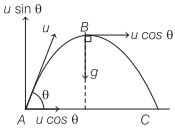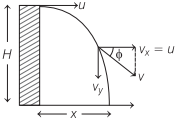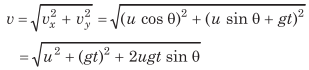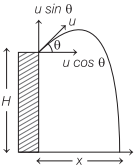# Projectile Motion | Definition, Equations, Formulas, Types – Motion in a Plane

Projectile Motion Physics
When any object is thrown from horizontal at an angle θ except 90°, then it moves on a parabolic known as its trajectory, the object is called projectile and its motion is called projectile motion.

We are giving a detailed and clear sheet on all Physics Notes that are very useful to understand the Basic Physics Concepts.

## Projectile Motion | Definition, Equations, Formulas, Types – Motion in a Plane

If any object is thrown with velocity u, making an angle θ, from horizontal, thenHorizontal component of initial velocity = u cos θ.
Vertical component of initial velocity = u sin θ.
Horizontal component of velocity (u cos θ) remains same during the whole journey as no force is acting horizontally.
Vertical component of velocity (u sin θ) decreases gradually and becomes zero at highest point of the path.
At highest point, the velocity of the body is u cos θ in horizontal direction and the angle between the velocity and acceleration is 90°.

Time of Flight of Projectile
It is defined as the total time for which the projectile remains in air.
$$T=\frac{2 u \sin \theta}{g}$$

Maximum height of Projectile
It is defined as the maximum vertical height covered by projectile.
$$H=\frac{u^{2} \sin ^{2} \theta}{2 g}$$

Horizontal range of Projectile
It is defined as the maximum distance covered in horizontal distance.
$$R=\frac{u^{2} \sin 2 \theta}{g}$$

Important Points and Formulae of Projectile Motion
(i) At highest point, the linear momentum is mu cos θ and the
kinetic energy is $$\frac{1}{2}$$ m(u cos θ)².

(ii) The horizontal displacement of the projectile after t seconds,
x = (u cos θ) t

(iii) The vertical displacement of the projectile after t seconds,
y=(u sin θ)t-$$\frac{1}{2}$$gt²

(iv) Equation of the path of projectile,
$$y=x \tan \theta-\frac{g}{2 u^{2} \cos ^{2} \theta} x^{2}$$

(v) The path of a projectile is parabolic.

(vi) Velocity of the projectile at any instant t,
$$|\mathbf{v}|=\sqrt{u^{2}+g^{2} t^{2}-2 u g t \sin \theta}$$

(vii) Kinetic energy at the lowest point = $$\frac{1}{2}$$ mu²

(viii) Linear momentum at lowest point = mu
(ix) Acceleration of projectile is constant throughout the motion and it acts vertically downwards being equal to g.
(x) Angular momentum of projectile =mu cos θ x h, where h denotes the height.
(xi) In case of angular projection, the angle between velocity and acceleration varies from 0° < θ < 180°.
(xii) The projectile attains maximum height when it covers a horizontal distance equal to half of the horizontal range, i.e. R/2.
(xiii) When the maximum range of projectile is R, then its maximum height is R/4.

• Horizontal range is maximum when it is thrown at an angle of 45° from the horizontal
$$R_{\max }=\frac{u^{2}}{g}$$
• For angle of projection θ and (90° – θ), the horizontal range is same.

Projectile Projected at an Angle θ with the Vertical
Let a particle be projected vertically with an angle θ with vertical and speed of projection is uTime of Flight, $$T=\frac{2 u \sin \left(90^{\circ}-\theta\right)}{g}=\frac{2 u \cos \theta}{g}$$
Maximum height, $$H=\frac{u^{2} \sin ^{2}\left(90^{\circ}-\theta\right)}{2 g}=\frac{u^{2} \cos ^{2} \theta}{2 g}$$
Horizontal range, $$R=\frac{u^{2} \sin \left(180^{\circ}-2 \theta\right)}{g}=\frac{u^{2} \sin 2 \theta}{g}$$

Equation of path of projectile, y = x cot θ – $$\frac{g x^{2}}{2 u^{2} \sin ^{2} \theta}$$

Projectile Projected from Some Height

1. When Projectile Projected HorizontallyInitial velocity in vertical direction = 0

Time of flight, T = $$\sqrt{\frac{2 H}{g}}$$
Horizontal range, x = uT = $$u \sqrt{\frac{2 H}{g}}$$
Vertical velocity after t seconds,
vy = gt (∵uy = 0)
Velocity of projectile after t seconds,
v = $$\sqrt{v_{x}^{2}+v_{y}^{2}}=\sqrt{u^{2}+(g t)^{2}}$$

If velocity makes an angle Φ from horizontal, then
tan Φ = $$\frac{v_{y}}{v_{x}}=\frac{g t}{u}$$
Equation of the path of the projectile,
$$y=\frac{g}{2 u^{2}} x^{2}$$

2. When Projectile Projected Downward at an Angle θ with HorizontalInitial velocity in horizontal direction = u cos θ
Initial velocity in vertical direction = -u sin θ
Time of flight, $$T=-\frac{2 u \sin \theta}{2 g} \pm \frac{\sqrt{4 u^{2} \sin ^{2} \theta+8 g h}}{2 g}$$
Horizontal range, x=(u cos θ) T
Vertical velocity after t seconds,
vy = u sin θ + gt
Velocity of projectile after t seconds,3. When Projectile Projected Upward at an Angle θ with HorizontalInitial velocity in horizontal direction = u cos θ
Initial velocity in vertical direction = u sin θ
Time of Flight, $$T=\frac{u \sin \theta}{g} \pm \sqrt{\frac{u^{2} \sin ^{2} \theta}{g^{2}}+\frac{2 h}{g}}$$
Horizontal range, x=(u cos θ)T
Vertical velocity after t seconds, vy = (- u sin θ) + gt
Velocity of projectile after t seconds,Projectile Motion on Inclined Plane Formulas
When any object is thrown with velocity u making an angle α from horizontal, at a plane inclined at an angle β from horizontal, thenInitial velocity along the inclined plane = u cos (α – β)
Initial velocity perpendicular to the inclined plane = u sin (α – β)
Acceleration along the inclined plane = g sin β
Acceleration perpendicular to the inclined plane = g cos β
Time of flight, $$T=\frac{2 u \sin (\alpha-\beta)}{g \cos \beta}$$
Maximum height, $$H=\frac{u^{2} \sin ^{2}(\alpha-\beta)}{2 g \cos \beta}$$
Horizontal range, $$x=\frac{2 u^{2} \sin (\alpha-\beta) \cos \alpha}{g \cos \beta}$$
Range on inclined plane,
$$R=\frac{x}{\cos \beta}=\frac{2 u^{2} \sin (\alpha-\beta) \cos \alpha}{g \cos ^{2} \beta}$$
Range on inclined plane will be maximum, when
α = 45° + $$\frac{\beta}{2}$$
$$R_{\max }=\frac{u^{2}}{g(1+\sin \beta)}$$
For angle of projections a and (90° – α + β), the range on inclined plane are same.
If the projectile is thrown downwards, then maximum range is
$$R_{\max }=\frac{u^{2}}{g(1-\sin \beta)}$$

Motion in a Plane (Projectile and Circular Motion):
In this chapter or under this topic, we are going to come across the motion of the object when it is thrown from one end to another end. This practice is said to be projection. Also, when an object is moved in a circular motion, then the equation of the motion is derived here. We will learn here about centripetal force and centripetal acceleration in detail with formulas. Also learn the force applied in everyday life motion of the particle in a vertical circle.